## Example Questions

### Example Question #8 : Inequalities

The cost, in cents, of manufacturingpencils is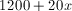, where 1200 is the number of cents required to run the factory regardless of the number of pencils made, and 20 represents the per-unit cost, in cents, of making each pencil. The pencils sell for 50 cents each. What number of pencils would need to be sold so that the revenue received is at least equal to the manufacturing cost?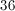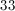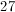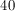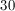Explanation:

If each pencil sells at 50 cents,pencils will sell at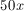. The smallest value ofsuch that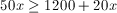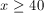### Example Question #11 : Inequalities

Find the slope of the inequality equation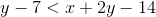0

–1

7

–7

1

–1

Explanation: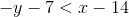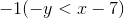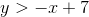From the equation we can see that the slope is –1.

### Example Question #12 : Inequalitiesandare both integers.

If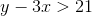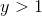, and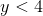, which of the following is a possible value of?Explanation:

Take the values of y that are possible, i.e. 2 and 3, and plug them into the first inequality. First, plug in 2. 2 – 3x > 21. Subtract 2 from both sides, and then divide by –3. Don't forget that when you divide or multiply by a negative number in an inequality you must flip the inequality sign. Thus, x < –19/3. Now plug in 3. We find, following the same steps, that when y=3, x < –6. Thus –7 is the correct answer.

### Example Question #13 : Inequalities

Quantity A:

The value(s) for which the following function is undefined: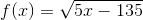Quantity B:Which of the following is true?

The two quantities are equal.

Quantity B is larger.

Quantity A is larger.

A comparison cannot be detemined from the given information.

Quantity B is larger.

Explanation:

This question is not as hard as it seems.  Remember that for real numbers, square roots cannot be taken of negative numbers.  Therefore, we know that this function is undefined for: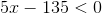This is simple to solve.  Merely add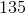to both sides: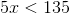Then, divide by: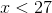Therefore, quantity A is less than quantity B.  This means that quantity B is greater than it.

### Example Question #14 : Inequalities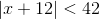Quantity A:Quantity B:Which of the following is true?

A comparison cannot be detemined from the given information.

The two quantities are equal.

Quantity B is larger.

Quantity A is larger.

A comparison cannot be detemined from the given information.

Explanation:

Recall that when you have an absolute value and an inequality like,

this is the same as saying that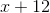must be between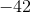and.  You can rewrite it: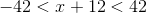To solve this, you merely need to subtractfrom all three values: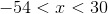Sinceis between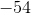and, it could be both larger or smaller than.  Therefore, you cannot determine the relationship based on the given information.

Tired of practice problems?

Try live online GRE prep today.